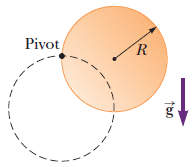# Uniform solid disk equations

## Homework Statement

A uniform solid disk of radius R and mass M is free to rotate on a frictionless pivot through a point on its rim (see figure below). The disk is released from rest in the position shown by the copper-colored circle.(a) What is the speed of its center of mass when the disk reaches the position indicated by the dashed circle? (Use any variable or symbol stated above along with the following as necessary: g.)

(b) What is the speed of the lowest point on the disk in the dashed position? (Use any variable or symbol stated above along with the following as necessary: g.)

(c) Repeat part (a) using a uniform hoop of mass M. (Use any variable or symbol stated above along with the following as necessary: g.)

## Homework Equations

KEf + Uf = KEi + Ui
1/2Iω2
Impulse for disk = 1/3MR2
Vcm = Rω
KE = 1/2MV2
U = mgR

## The Attempt at a Solution

Part A I got the right answer of $\sqrt{}4gR/3$

Part B is giving me some issues and I suppose I am not sure where to go with it.

Part C I figured I could use the same method as part A, but for some reason, I get $\sqrt{}2gR$ and that is no good.